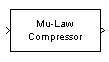# Mu-Law Compressor

Implement µ-law compressor for source coding

## Library

Source Coding

•## Description

The Mu-Law Compressor block implements a µ-law compressor for the input signal. The formula for the µ-law compressor is

`$y=\frac{V\mathrm{log}\left(1+\mu |x|/V\right)}{\mathrm{log}\left(1+\mu \right)}\mathrm{sgn}\left(x\right)$`

where µ is the µ-law parameter of the compressor, V is the peak magnitude of x, log is the natural logarithm, and sgn is the signum function (`sign` in MATLAB®).

The input can have any shape or frame status. This block processes each vector element independently.

## Parameters

mu value

The µ-law parameter of the compressor.

Peak signal magnitude

The peak value of the input signal. This is also the peak value of the output.

## Supported Data Type

PortSupported Data Types
In
• double

Out
• double

Mu-Law Expander

## References

 Sklar, Bernard. Digital Communications: Fundamentals and Applications. Englewood Cliffs, N.J.: Prentice-Hall, 1988.

## Version History

Introduced before R2006a